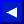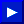The charts in Figure 3-3 explain sprite positioning.

By using these values, you can position each sprite anywhere. By moving the sprite a single dot position at a time, very smooth movement is easy to achieve.

### SPRITE POSITIONING SUMMARY

Unexpanded sprites are at least partially visible in the 40 column, by 25 row mode within the following parameters:

```                           1 < X < 343

30 < Y < 249
```
In the 38 column mode, the X parameters change to she following:
```                          8 <= X <= 334
```
In the 24 row mode, the Y parameters change to the following:
```                         34 <= Y <= 245
```

Expanded sprites are at least partially visible in the 40 column, by 25 row mode within the following parameters:

```                        489 >= X <= 343
9 >= Y <= 249
```
In the 38 column mode, the X parameters change to the following:
```                        496 >= X <= 334
```
In the 24 row mode, the Y parameters change to the following:
```                         13 <= Y <= 245
```This page has been created by Sami Rautiainen. Read the small print. Last updated May 12, 2002.